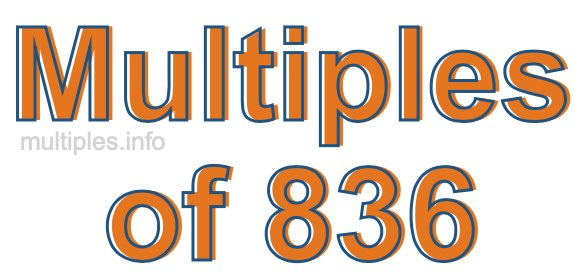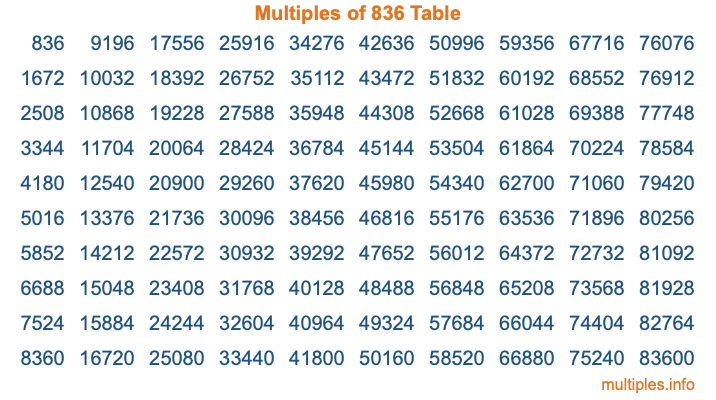Multiples of 836Welcome to the Multiples of 836 page. Here we will first teach you everything you will ever need to know about the multiples of 836, and then give you a study guide summary of everything we taught you to make sure you remember it all. Use this page to look up facts and learn information about the multiples of 836. This page will make you a multiples of eight hundred thirty-six expert!

Definition of Multiples of 836
Multiples of 836 are all the numbers that when divided by 836 equal an integer. Each of the multiples of 836 are called a multiple. A multiple of 836 is created by multiplying 836 by an integer.

Therefore, to create a list of multiples of 836, you start with 1 multiplied by 836, then 2 multiplied by 836, then 3 multiplied by 836, and so on for as long as you want. Thus, the list of the first five multiples of 836 is 836, 1672, 2508, 3344, and 4180. To see a larger list of multiples of 836, see the printable image of Multiples of 836 further down on this page. We also have a category where you can choose any nth multiple of 836.

Multiples of 836 Checker
The Multiples of 836 Checker below checks to see if any number of your choice is a multiple of 836. In other words, it checks to see if there is any number (integer) that when multiplied by 836 will equal your number. To do that, we divide your number by 836. If the the quotient is an integer, then your number is a multiple of 836.

Is  a multiple of 836?

Least Common Multiple of 836 and ...
A Least Common Multiple (LCM) is the lowest multiple that two or more numbers have in common. This is also called the smallest common multiple or lowest common multiple and is useful to know when you are adding our subtracting fractions. Enter one or more numbers below (836 is already entered) to find the LCM.

Check out our LCM Calculator if you need more details about the Least Common Multiple or if you need the LCM for different numbers for adding and subtraction fractions.

nth Multiple of 836
As we stated above, 836 is the first multiple of 836, 1672 is the second multiple of 836, 2508 is the third multiple of 836, and so on. Enter a number below to find the nth multiple of 836.

th multiple of 836

Multiples of 836 vs Factors of 836
836 is a multiple of 836 and a factor of 836, but that is where the similarities end. All postive multiples of 836 are 836 or greater than 836. All positive factors of 836 are 836 or less than 836.

Below is the beginning list of multiples of 836 and the factors of 836 so you can compare:

Multiples of 836: 836, 1672, 2508, 3344, 4180, etc.

Factors of 836: 1, 2, 4, 11, 19, 22, 38, 44, 76, 209, 418, 836

As you can see, the multiples of 836 are all the numbers that you can divide by 836 to get a whole number. The factors of 836, on the other hand, are all the whole numbers that you can multiply by another whole number to get 836.

It's also interesting to note that if a number (x) is a factor of 836, then 836 will also be a multiple of that number (x).

Multiples of 836 vs Divisors of 836
The divisors of 836 are all the integers that 836 can be divided by evenly. Below is a list of the divisors of 836.

Divisors of 836: 1, 2, 4, 11, 19, 22, 38, 44, 76, 209, 418, 836

The interesting thing to note here is that if you take any multiple of 836 and divide it by a divisor of 836, you will see that the quotient is an integer.

Multiples of 836 Table
Below is an image of the first 100 multiples of 836 in a table. The table is in chronological order, column by column. The first column has the first ten multiples of 836, the second column has the next ten multiples of 836, and so on.The Multiples of 836 Table is also referred to as the 836 Times Table or Times Table of 836. You are welcome to print out our table for your studies.

Negative Multiples of 836
Although not often discussed or needed in math, it is worth mentioning that you can make a list of negative multiples of 836 by multiplying 836 by -1, then by -2, then by -3, and so on, to get the following list of negative multiples of 836:

-836, -1672, -2508, -3344, -4180, etc.

Multiples of 836 Summary
Below is a summary of important Multiples of 836 facts that we have discussed on this page. To retain the knowledge on this page, we recommend that you read through the summary and explain to yourself or a study partner why they hold true.

There are an infinite number of multiples of 836.

A multiple of 836 divided by 836 will equal a whole number.

836 divided by a factor of 836 equals a divisor of 836.

The nth multiple of 836 is n times 836.

The largest factor of 836 is equal to the first positive multiple of 836.

836 is a multiple of every factor of 836.

836 is a multiple of 836.

A multiple of 836 divided by a divisor of 836 equals an integer.

836 divided by a divisor of 836 equals a factor of 836.

Any integer times 836 will equal a multiple of 836.

Multiples of a Number
Here you can get the multiples of another number, all with the same attention to detail as we did for multiples of 836 on this page.

Multiples of
Multiples of 837
Did you find our page about multiples of eight hundred thirty-six educational? Do you want more knowledge? Check out the multiples of the next number on our list!